Latest Banking jobs   »

# Quantitative Aptitude Quiz For RBI Grade B Phase 1 2023 – 12th May

Directions (1 – 5): The following table shows the total no. students and percentage of students present on a particular day out of them for two schools A and B in different classes.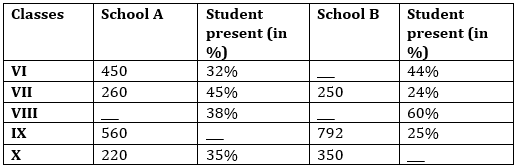Q1. If total number of students in class VIII in both the school together are 1625, while total students present in both the classes together are 766, then find difference between number of students in class VIII in both the schools?
(a)250
(b) 275
(c) 225
(d) 300
(e) None of these

Q2. The number of students present in class VI in school A are approximately what percent of number of students present in class IX in school B?
(a)64%
(b) 96%
(c) 52%
(d) 73%
(e) 81%

Q3. If number of students present in class VI in school B are 2 less than double of the number of students presents in same class in school A, then find the total number of students in class VI in school B.
(a)625
(b) 650
(c) 600
(d) 700
(e) None of these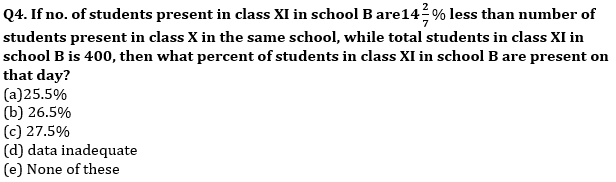Q5. Find the total number of students present in class VII in both the schools together?
(a) 157
(b) 187
(c) 167
(d) 177
(e) None of these

Directions (6-10) :- Table given below gives information about percentage of quantity of milk sold by Vikash to different persons i.e. (P, Q, R, S and T). Vikash has 1000 lit of pure milk out of which 60% are sold to these persons. Each person then adds some quantity of water to it and again replaces an amount of mixture with the equal amount of water. Some data is missing. Students are supposed to calculate the data and answer the questions that follows.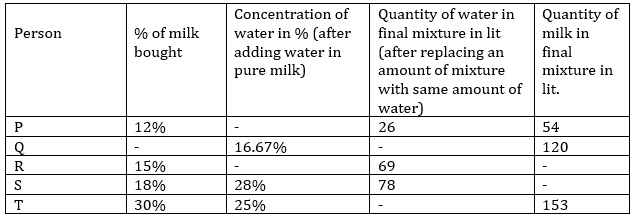Q6. Quantity of water initially added in pure milk by S is what percent more or less than quantity of mixture replaced with water by P.
(a) 105%
(b) 110%
(c) 120%
(d) 80%
(e) 70%

Q7. If quantity of milk in final mixture for person R is 12.5% more than that of person S, then find amount of water added by R initially in pure milk.
(a) 45 lit.
(b) 50 lit.
(c) 60 lit.
(d) 75 lit.
(e) 40lit.

Q8. Find quantity of water in the final mixture of Q.
(a) 30 lit.
(b) 50 lit.
(c) 45 lit.
(d) 60 lit.
(e) 40 lit.

Q9. Cost price of pure milk is Rs. 200 per lit, then find approximate profit percent earned by T if he sells final mixture of water and milk at cost price of pure milk. Consider water is freely available.
(a) 66%
(b) 46%
(c) 44%
(d) 54%
(e) 48%

Q10. Find the ratio of quantity of milk sold by Vikash to another person A(quantity of milk sold by Vikash to another person A is 20% more than quantity of pure milk sold to S) to quantity of pure milk remained with Vikash after selling to six different persons?
(a) 81/169
(b) 64/169
(c) 16/35
(d) 35/78
(e) 75/169

Solutions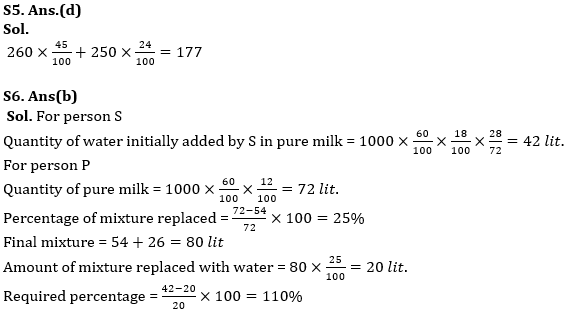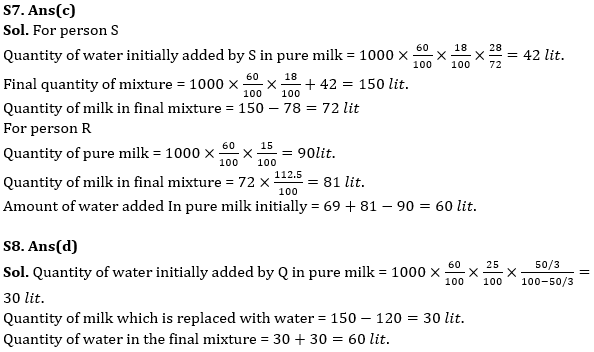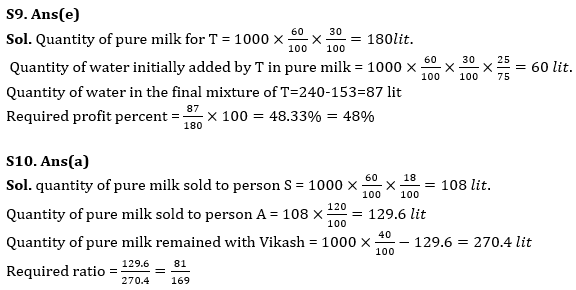## FAQs

### How many sections are there in the RBI Grade B Phase 1 Exam?

How many sections are there in the RBI Grade B Phase 1 Exam?

#### Congratulations!Union Budget 2023-24: Free PDF